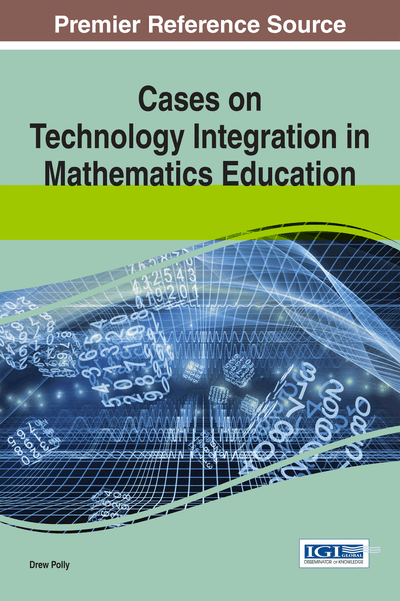# Leveraging Dynamic and Dependable Spreadsheets Focusing on Algebraic Thinking and Reasoning

Margaret L. Niess (Oregon State University, USA)
DOI: 10.4018/978-1-4666-6497-5.ch001
Available
\$37.50
No Current Special Offers

## Abstract

Algebraic thinking and reasoning are important skills for students to develop as they transition from arithmetic to algebra. The Common Core State Standards for Mathematics links these ideas through their mathematical practices where students make sense of mathematical problems, reason abstractly, model with mathematics, and use appropriate tools that engage them in algebraic thinking and reasoning. This chapter focuses on how a specific tool—the spreadsheet—supports students in building their understandings of spreadsheet features and capabilities while also engaging them in thinking more logically about numbers as needed in preparation for formal algebra. The challenge for teachers is to scaffold students' learning about and with spreadsheets as mathematics learning tools for exploring patterns, variables, and linear problems. This chapter describes this transition through multiple problems where students learn to design dynamic and dependable spreadsheets in ways that concurrently develop their algebraic reasoning and thinking through middle grades mathematics problems.
Chapter Preview
Top

## Introduction

What is algebra? A student response is often “Arithmetic with letters.” Keith Devlin (2011), a Stanford mathematician, however, characterizes algebra much differently. He describes algebra as a way of thinking that involves reasoning logically about numbers rather than thinking with numbers as in arithmetic. From this perspective, algebraic reasoning is a way of thinking when learning algebra. In fact, students benefit from mathematics programs focused on building a strong foundation in algebraic thinking and reasoning prior to the formal algebra program (Carraher, Schliemann, Brizuela & Earnest, 2006; Nathan & Koedinger, 2000). Exploring patterns, relations and functions, making generalizations and analyzing change are essential experiences for engaging students in algebraic reasoning.

The NCTM Principles and Standards (2000) talks about understanding patterns, analyzing mathematical situations, using mathematical models and analyzing change as keys to algebraic reasoning. The Common Core State Standards for Mathematics (CCSS-M, 2010) links these ideas through their eight mathematical practices (MP) in which the students need to be involved throughout the K-12 mathematics curriculum:

• MP1: Make sense of problems

• MP2: Reason abstractly

• MP3: Model with math

• MP4: Use appropriate tools

• MP5: Attend to precision

• MP6: Look for and make use of structure

• MP7: Look for and express regularity

• MP8: Construct viable arguments

These practices ultimately foster and support algebraic reasoning and thinking. The standards for every grade from kindergarten through fifth grade include a domain titled “Operations and Algebraic Thinking.” By the middle grades, the CCSS-M suggests that students are now ready to begin the study of algebra in a more abstract and symbolic way where they are guided in thinking logically about numbers.

Mathematics teachers in the middle grades are charged with identifying and incorporating ‘appropriate tools’ (MP4) for ‘math modeling’ (MP3) where students learn to think algebraically rather than continuing to think arithmetically. Devlin (2011) proposes the computer spreadsheet as the appropriate tool for this challenge: “With a spreadsheet, you don’t need to do the arithmetic; the computer does it, generally much faster and with greater accuracy than any human can. What you, the person, have to do is create that spreadsheet in the first place. The computer can’t do that for you” (para. 17).

Expand this vision of modeling with spreadsheets to the idea of incorporating dynamic and dependable spreadsheets as a key ingredient for algebraic thinking and reasoning. Dynamic and dependable spreadsheets are viewed as stimulating algebraic thinking and reasoning while algebraic thinking and reasoning animate the spreadsheet, such that the three are interactively portrayed in Figure 1.

## Complete Chapter List

Search this Book:
Reset